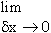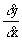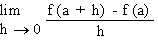Home MonkeyNotes Printable Notes Digital Library Study Guides Study Smart Parents Tips College Planning Test Prep Fun Zone Help / FAQ How to Cite New Title Request

CHAPTER 4 : DERIVATIVES

In this chapter we shall study the derivative, its existence and applications. We shall be concerned mainly with the real valued functions of a real variable i.e. the domains and ranges of the functions considered here will be sets of real numbers.

4.1 Derivability At A Point

Definition :

If a function  f  is defined by y = f(x) at a point (x,f (x) ) then, when it exists, is called the derivative of  f  at x and this derivative is symbolically denoted  byoror f (x) or y or Df when f  (x) exists, we say that  f  is derivable
(differentiable ) at x (figure. 1)Your browser does not support the IFRAME tag.if the limit exists.
The notation dx represents a small change in x.
Now f (x + dx) - f (x) represents the difference between two values of y = f (x) viz f (x + dx) and f (x) and Is denoted by dyThis is called the Leibnitzs notation and the detailed definition is as follows.

"If y is a function of x and when x changes to x + dx, correspondingly y changes to y + dy, then the value of the limit,, if it exists, is called the derivative of y with respect to x " and is denoted by.

The notationis widely used in all practical work involving derivatives. It is especially used in proving theorems of derivatives.

In particular, the derivative of f at x = a of its domain, is defined by
f  (a) =or byIndex

4. 1 Derivability At A Point
4. 2 Derivability In An Interval
4. 3 Derivability And Continuity Of A Function At A Point
4. 4 Some Counter Examples
4. 5 Interpretation Of Derivatives
4. 6 Theorems On Derivatives (differentiation Rules)
4. 7 Derivatives Of Standard Functions
4. 8 Derivative Of A Composite Function
4. 9 Differentiation Of Implicit Functions
4.10 Derivative Of An Inverse Function
4.11 Derivatives Of Inverse Trigonometric Functions
4.12 Derivatives Of Exponential & Logarithmic Functions
4.13 Logarithmic Differentiation
4.14 Derivatives Of Functions In Parametric Form
4.15 Higher order Derivatives

Chapter 5